A new idea to obtain clean fusion energy

11. Confinement of positive ions and electrons with a static electric and magnetic field

Main page Calculations     (Explained in chronological order, how I myself discovered everything step by step....)

The goal is to design a kind of fusor ( ). But instead of a fixed inner grid, which causes losses, we try to confine  positive H+ and B+ ions with a static electric field and a static magnetic field.

We'll use our computer simulation program, as described in:  10b computer simulation
(by the way: also other type of ions can be used, for example deuterium ions)

Let us simulate the following device:

Fig. 1. Schematic representation of a Static Electro Magnetic fusion device (S.E.M. fusor?, Sem ).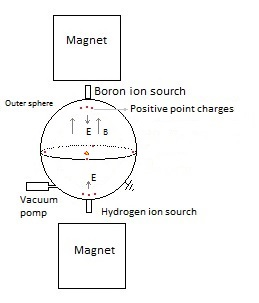The sphere (vacuum chamber) has been pumped vacuum and is earthed.

Above and under the sphere (or around) a magnet is placed which generates a vertical magnetic field inside the sphere.  We'll try out several magnetic field configurations in the computer program.

An electric field is created by placing eight point charges: four near the top and four near the bottom. In a real apparatus this could be done different (perhaps with rings? see applet), but in order to simulate it easily in our computer program we'll do it at the moment in this way (with point charges). In the middle of each side is also a point charge placed.

Fig. 2.   The simulation space, with four positive point charges above and four under ( and one in each side; not in the drawing).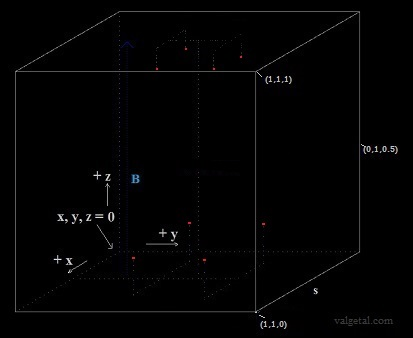Several experiments are performed with the simulation program, see:  11.1 Experiments 11.1 till 11.6 and Video Youtube

The ions have a random initial speed up to 0,5E6 m/s in all directions.

Potential of an electrical charged sphere: V= 9E9. Q /r    (r=radius of sphere, Q=charge of sphere, V=electrical potential of sphere)
A (solitary) sphere with diameter 10 cm and a voltage of +1kV (to infinity) has a charge of: qi= 1000/(9E9)* 0.05 = 5.56E-09 coulomb.
100 kV -> 5.56E-7  coulomb.
A (solitary) sphere with diameter 5 cm and a voltage of +2kV (to infinity) has a charge of: qi= 2000/(9E9)* 0.025 = 5.56E-09  coulomb.
200 kV -> 5.56E-07 coulomb
.

The movement of the positive ions is simulated  using  Leap-frog integration (see 10.1 experiment10 ). The ions interact amongst themselves and with the electric and magnetic field applying the Coulomb and Biot-Savart formulism ( non-relativistic).

 Conclusions so far.If we use a constant magnetic field of about 1 or 2 T (should be possible to realize..), and a constant electric field generated by the point charges as described before, the positive ions are confined in the simulation space. Even if we give them an initial speed up to 0,5E6 m/s in all directions. At least they are confined during the (very) short time of simulation. The magnetic field is important: if it is decreased the ions escape away to the sides. No interactions (collisions) between the ions are observed. The reason of this  is, probably, because the ions are relatively very small and there are only a very few (in reality there would be millions..). When the charge of the ions is increased a 10000000 times, then yes interactions between them are observed. I let one H+ and one B+ ion collide with each other. If the charge is increased 100000 times  and dt= 1.59E-14, then they did not collide  With dt=1.59E-15 and  100000 times more charge they do collide. (dt = Δt = the integration time, see 10.1 experiment10 ) So far so good....  Could this be a fusor without inner grid?   I did a search in internet, but could not find anything similar.. It should be almost impossible that this simple idea has not been tried out already..... However, I got this idea because I have my program, with which I can try out easily different kind of configurations. It took me quite a long time to develop it; could it be that I am the only one so far with a simulation program like this?Perhaps there is some error in the software, perhaps it works only with a few ions (and not with millions) and with non relativistic  formulas; perhaps it works only for the very short simulation time (about 3E-5 s) ; perhaps the ions stay confined but will not fuse....   (in a fusor with inner grid they move towards the centre; here they move "more or less" towards the centre). Perhaps the accelerating and decelerating of the ions causes too many losses.. (bremstrahlung). But until I have proof that it cannot produce net energy, I should continue, because there could be a small change that it does work.... Anyway it's fun to work on it ☺

To check the correctness of the simulation, we will calculate the total energy of all particles.

The kinetic energy of all moving particles is calculated (electrons, H+ and B+ ions).
The potential energy between H+-H+,  H+-B+,  H+-point charges,  B+-B+, etc. is calculated.
When the potential energy between equal particles is calculated in two loops, in the end we divide by 2, see also 10.9 experiment9 .
Note: also the potential energy of the moving particles with respect to the fixed charges is calculated. The potential energy of the fixed charges amongst themselves is not calculated (is constant).

Part of the program:

 for i:=1 to (AmountB) do begin //*  xi:=boron[i].x;  yi:=boron[i].y;  zi:=boron[i].z; f or j:=1 to AmountH do  begin   xj:=hydrogen[j].x;   yj:=hydrogen[j].y;   zj:=hydrogen[j].z; {K is Coulomb's constant, see unitB}   r:=sqrt( sqr(xi-xj)+sqr(yi-yj)+ sqr(zi-zj) );   if boron[i].exists and hydrogen[j].exists then Ue:= Ue+ K*qe*qe/r; {neither we have to divide by 2, because we do not calculate double pairs like we did with the electrons} end; end; //*

The positive charge of each fixed point charge is:  5.56E-7 Q (100 kV for a sphere with diameter 10 cm), the magnetic field constant 1 tesla, generated 200 H+ and 200 B+ with initial random speed < 0,5E6  m/s. With dt=1E-9 s no ions escaped and the total energy of the particles stayed constant.

 Conclusion: The total energy of the moving particles stayed constant (within limits) and they stayed confined in the simulation space. Good!  ☺

When positive charged ions collide, some of them can get higher speeds and could escape. This could perhaps be prevented by two charged grids, as shown in figure 3:

Fig. 3. Schematic representation of an electrostatic magnetic fusion device with two grids near the outer wall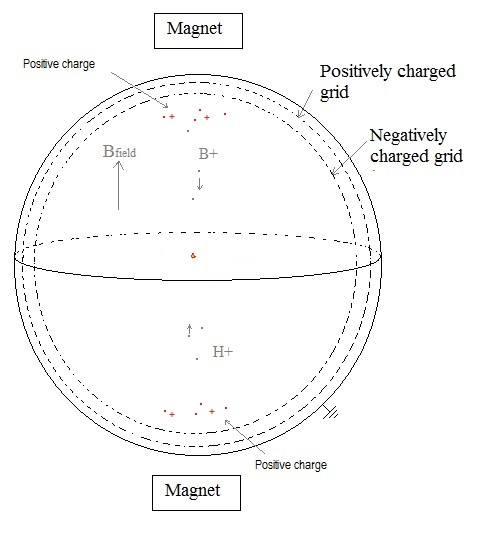The negatively charged grid must be close enough, so it does not produce a "negative" electric field inside the sphere. But also it must be open enough (with holes), to prevent that too much positive ions collide with it, producing unwanted losses.

Our simulation space is a cube with a side of 1 m.
Let's take the magnetic field strength 1 tesla.
What is the maximum horizontal speed of the ions so that r <= 0,5 m? (non relativistic)

Fig. 4. Moving H+ ion in a magnetic field B.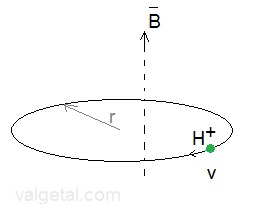massa boron ion (m) = 11 . 1,67E-27 kg charge B+  (q)  = 1,60E-19  coulomb B-field (B) = 1,00 tesla B = m.v / (r.q) r=0,5 m -> v = r.B.q / m = 0,5 . 1,00 . 1,60E-19  / (11 .1,67E-27) = 4,35E6 m/s r=0,7 m -> v = 6,10E6 m/s massa deuterium ion(m) = 2 . 1,67E-27 kg charge D+  (q)  = 1,60E-19  coulomb B-field (B) = 1,00 tesla B = m.v / (r.q) r=0,5 m ->  v = r.B.q / m = 0,5 . 1,00 . 1,60E-19  /    (2 . 1,67E-27) = 2,4E7 m/s r=0,7 m -> v = 3.3 E7 m/s massa hydrogen ion  (m) = 1,67E-27 kg charge H+  (q)  = 1,60E-19  coulomb B-field (B) = 1,00 tesla B = m.v / (r.q) r=0,5 m ->  v = r.B.q / m = 0,5 . 1,00 . 1,60E-19  /    (1,67E-27) = 4,8E7 m/s r=0,7 m -> v = 6,7 E7 m/s

According to https://en.wikipedia.org/wiki/Fusor#Basic_fusion, for fusion to occur the ions must be at a temperature of at least 4 keV (kiloelectronvolts) or 45E6 K.
Assuming this refers to deuterium and tritium, that are easiest to fuse. But let's take this temperature just to get an idea.
4 keV = 4E3 . 1,6E-19 J = 6,4E-16 J = 0,5 . m . v2 .   m (H+) = 1,67E-27 kg   m(B+) = 11.1,67E-27 kg
-> v = (6,4E-16 . 2 /m )    H+ -> v = 8,8 E5 m/s   B+ -> v = 2,6 E5 m/s
T = 45E6 K, mD+ =2.1,67E-27, ½mv2 = 3/2.k .T
(see below) -> v = ( 3.k.T/ mD+) = 7,5 E5 m/s

We look a little further:

In  euro-fusion.org  a temperature of about 450E6  degrees C is /was) mentioned for D-D fusion reaction to occur.
In hyperphysics a temperature of  40E7 K is mentioned for D-D fusion.
According Wikipedia..electronvolt  1 degree is about 8,8E-5 eV , so  450E6 . 8,8E-5  = 39.6 keV
Now the needed temperature is ten times higher:
-> v = (6,4E-15 . 2 /m )    H+ -> v = 2.8 E6 m/s   B+ -> v = 8.3 E5 m/s  D+ -> 2E6 m/s
In hyperphysics with ½mv2 = 3/2.k .T
(k=Boltzmann constant) we get about the same speed.

(Boltzmann constant = 1.38064852 × 10-23 m2 kg s-2 K-1)

Also in hyperphysics it is suggested that the currently attainable particle energies in thermonuclear reactors is in the range 1-10keV, corresponding to 0.77E7 K- 0.77E8 K. Filling in 0.77E8 K in  hyperphysics   we get v 1E6 m/s (for a deuterium ion).

These temperature-speed calculations are not very correct/detailed, but anyway we get some idea about it.

It seems to be that with a magnetic field of 1 tesla we could more or less confine  D+ particles up to about 2,4E7 m/s. This is ten times higher than the speed needed to fuse ... So the confinement to the sides seems to be okay.

In the simulation program we gave the ions an initial random speed in all directions of 0.5 E6 m/s . This is 25 % of the speed needed for D+ to fuse. If we increase this initial speed, some particles escape upwards and downwards (with B=1 T and 200 kV).

 Conclusion: The problem is to confine the fastest particles upwards and downwards. Increasing the B-field and/or potential is not very attractive.. Perhaps we could place more point charges up and down. We will investigate this further..

We increased the electric field by creating more positive point charges above and increasing the voltage to 300 kV; applied a magnetic field of 1,5 tesla; generated 20 H+ and 20 B+ ions with an initial speed up to 1,5E6 m/s in all directions, and the ions stayed ... confined!

Instead of hydrogen and boron, we will use now only deuterium ions. Massa D+ = 2 x massa H+ . B=1 tesla and 200 kV,  initial speed up to 1,5E6 m/s. The ions stayed confined. Also with B=0,7 tesla and 90 kV they stayed confined.

 According to experiment 11.7 it seems to be possible to confine positive H+ and B+ ions with random speeds in all directions up to 1,5E6 m/s,  in a reasonable (realizable) constant magnetic field of 1,5 tesla and in a reasonable constant electric field . (I assume the electric field is realizable... ). In experiment 11.8 we used only D+ ions (30 particles,  also with random speeds in all directions up to 1,5E6 m/s). With B=1 tesla and 200 kV (potential of the point charges if they would be little spheres with radius 5 cm). The ions stayed good confined. In experiment 11.9 we used D+ ions (30 particles,  random speeds in all directions up to 1,5E6 m/s). With B=0,7  tesla and 100 kV (potential of the point charges if they would be little spheres with radius 5 cm).  The ions stayed also good confined. Deuterium is what is usually used in fusors. So far, ever better...

For fusion to occur, it seems to be necessary to have an amount of 10^18 ions  per cubic meter= 1E18 . 1,6E-19 C = 1,6 C ( a lot of charge).
If it is mixed with electrons, the net charge will be less. But what do these electrons?

 In the program we use positive point charges. In reality these will be conductors. The positive charge will move to the outside and this will decrease the E-field in the inside of the vacuum chamber...? See the screenshot  of an applet in fig. 8b. This is not good ... I shall have to simulate positive charged conductors, place them in the vacuum chamber and see what happens. Or would it be possible to charge a non-conducting object, which then can be considered as a point charge?

9,4 ltr diesel, see energy_conversion_calculator   1 J = 2,6E-8 ltr diesel )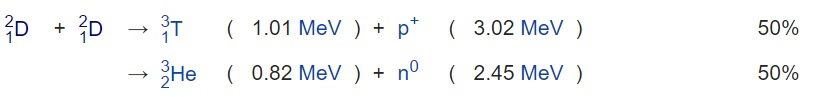( see: Wikipedia )

Per ion about 3,7/2 MeV is liberated = 1,8E6 . 1,6E-19 J  = 2,9E-13 J
100 kWh = 100E3 x3600 J = 3,6E8 J
-> 3,6E8 / 2,9 E-13 = 12,3 E20 ions.
1 ion D+ = 2 .  1,67E-27 kg

100 kWh -> 12,3E20 ions -> 4,1E-6 kg = 4,1 E-3 gram of deuterium (1 gram deuterium = 8,78E10 J =2283 ltr diesel).

---

To get an idea about the charge:

100 W = 100 J/s -> 3,4E14 ions/s

3,4E14 ions = 3,4E14 . 1,6E-19 = 5,5 E-5 coulomb  -> (5,5E-5)/ (5.56E-7) =>  charge of  198 small spheres with  diameter 5 cm & 200 kV

Let's imagine two spheres, each sphere with half this charge, at a distance of 10 cm: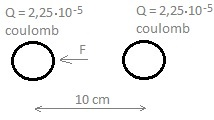They will exert a force on each other: F = 9.109 . 2,25.10-5 . 2,25.10-5  / 0,12 = 455,6 N 46 kgf

200kV x  5,5 E-5 C/s = 11 W

---

 If we use for the up and down positive charges a ring, the electrons are attracted to the ring, but fly through it and will move up and down.. I see this happening in the simulation if I generate electrons. Perhaps an idea. If I charge the point charges in the middle of the vertical side negative, the ions keep trapped. This is quite similar to the already existing Penning trap: Wikipedia./Penning_trap . I did not know about this, just invented it more or less again. They got the Nobel Price for it..... The problem of this Penning trap is (according my opinion) that it can confine only ions of one type (or positive, or negative), and only a few. Would it be possible to modify it to trap both electrons and positive deuterium ions?

We will put some negative point charges in the centre of the top and the bottom side.

 The electrons and deuterium ions stayed more or less confined, although some D+ ions escaped.

I moved the negative point charges in the top side 20 cm upwards, and the ones in the bottom side 20 cm downwards.

D+ ions  and electrons got an initial random speed < 1,5E6 m/s  (about 2E6 is needed for fusion) .

 The electrons and deuterium ions stayed confined!

How we could give this simulation shape in the real world?

For the negative charges above and bellow we could perhaps use ellipsoidal metal conductors, for the negative charges in the sides a metal ring and for the positive charges two metal rings:

Fig. 5. Possible device that corresponds with the simulation (S.E.M. fusor).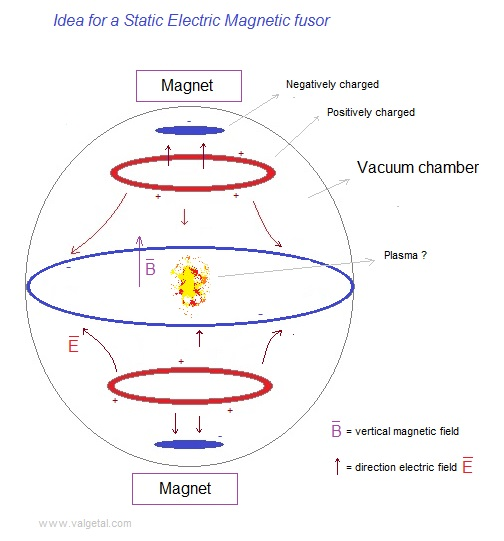Cross section of the electric field according www.falstad.com.

Possible  modus operandi:

The vacuum chamber is pumped empty. Only some deuterium gas is discharged inside. (The best way to do this should be investigated: perhaps most favourable is to inject it along the vertical centre line.) This gas is ionized (I suppose) into D+ ions and electrons because of the electric field .

The electrons (more or less near the vertical centre line) are attracted by the two positively charged rings and move upwards and downwards in a spiral-shaped path. The vertical magnetic field of 1 tesla prevents them to escape sideward. Once they move through the ring they will be repelled by the negatively charged ellipsoid and move back towards the centre. In this way the electrons will oscillate between the blue ellipsoids and the centre of the vacuum chamber. (this is what happens in the simulation)

The D+ ions (more or less near the vertical centre line) are repelled by the two positively charged rings and will vertically oscillate around the centre of the vacuum chamber, also in a spiral-shaped path. (this is what happens in the simulation)

In a "traditional" fusor the positive ions move towards the centre (a point). However, only when they are outside the inner grid they feel the force towards the centre. Once they are inside the inner grid (and they have not collided with it), they will not feel this force any longer (if I am not wrong).

In our S.E.M. fusor the D+ will oscillate in a vertical movement (al least in the simulation..). Will the same happen as in a traditional fusor: the positive D+ ions are confined and  attracted towards the centre; they collide between each other; some of them get as a result of these collisions higher speeds; some ions with higher speeds collide between each other and fuse..... ?

In the simulation there are only a few particles (it is not possible to have more than a couple of hundreds, otherwise it's takes too much memory of the computer and the simulation speed becomes too small).

In the program it is possible to turn on and off the interaction between the particles.  When there are only a few particles, it hardly influences the speed of the simulation, but when there are more particles, more then 100, then the simulation is running faster with the interaction turned of.

In the real world  possibly a cloud of electrons and deuterium ions (mixed together: a plasma),  will be confined more or less in the centre of the vacuum chamber.

Maybe we should construct this new type of fusor, and see what happens..

At the moment I will continue with the simulation program: visualize the electric field, simulate charged ring and ellipsoid conductors , do more experiments..

11.2 Various calculations etc (Cartesian and spherical coordinates, conducting surrounding sphere  i.a.)

14/1/17: there is now a button to choose whether yes or not to have a surrounding conducting sphere

11.1 Experiment 11.11
No conducting sphere, 100 D+ & 100 e-, ±160 kV, B= 1 tesla, 6,1E-6 sec, dt:=1E-9, 1E-10. 1E-11 s
All particles confined!

If the point charges in the sides are positive, the D+ ions escape upwards and downwards.
If the point charges in the sides are negative, the D+ ions stay confined.

Two positive rings, one negative ring and two negative charged spheres have been programmed, see:
11.3 More calculations (rings and spheres)

Fig. 6. The simulation space in experiment 11.14a.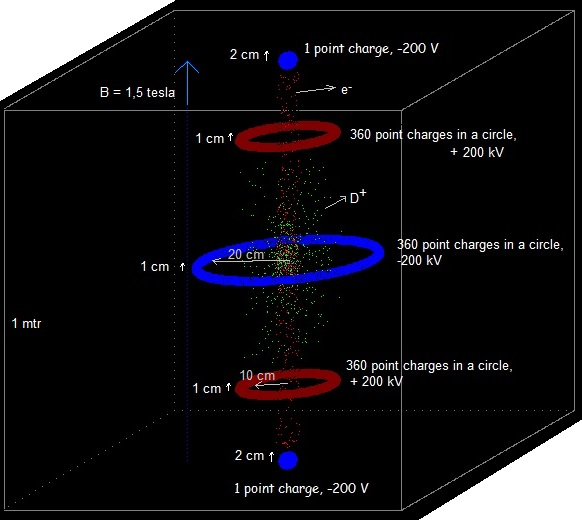11.14 experiments 11.14..19

With an initial random speed < 3E6 m/s (!) the D+ and the e- kept confined in the simulation space (during the short simulation time).

Although the simulation had been running about 48 hrs, the real simulation time is very small: 1,2E-5 s.
What I fear is that the particles will spread out sidewards after a larger time period.
I will program the maximum distance r from the vertical centre of the particles and see if it increases.
It did not seem to increase during some experiments I did.

The voltages can also be reversed: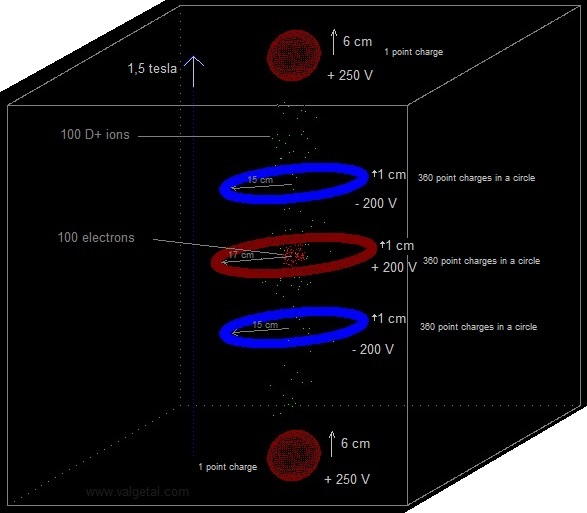The electrons are now more confined in the centre, but the D+ ions  spread more out in the vertical direction, although they do not escape (during the short simulation time). The D+ ions seem to obtain more speed in this configuration (= higher temperature). This would be an advantage to obtain fusion..  Furthermore, the electrons perhaps form a kind of virtual cathode that will attract the D+ ions towards the centre.

Fig. 7b.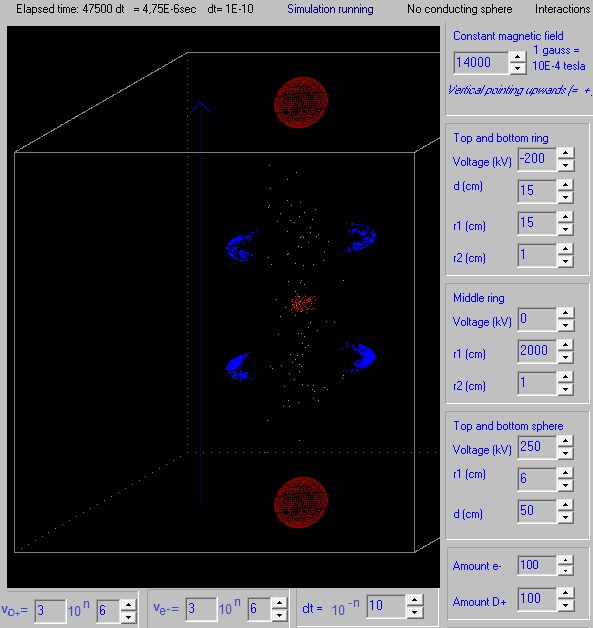In the experiment of figure 7b. the positive middle ring has been deleted. The electrons still stay confined and the D+ ions will obtain an higher speed in the centre (I suppose) compared with the configuration of figure 7a. Higher speed (is higher temperature) means more change of nuclear fusion.. However the confinement of the electrons is less compared with the configuration with a middle ring.

Fig. 8. Another drawing of  a possible design of the SEM fusor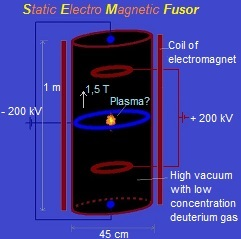Searching for similar already existing devices:

11.4 About creating the magnetic field

Fig. 9. Generating more than 5000 electrons (exp. 11.18)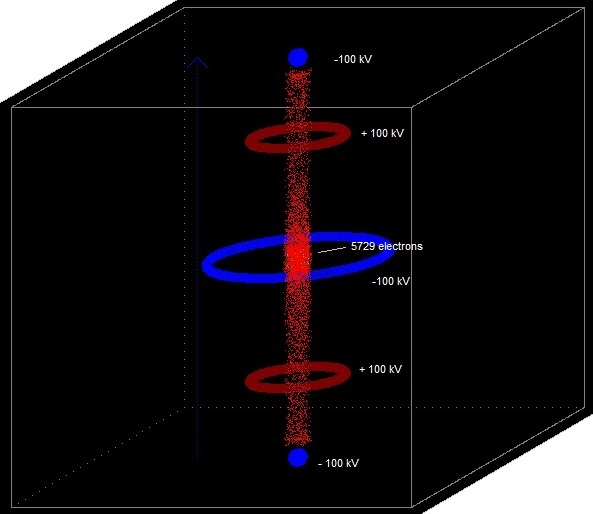The simulation program got stuck (did not proceed any longer) with this amount of particles.
What can be seen is that the electrons seems to concentrate near the centre. Perhaps in a real device they would form a kind of virtual cathode and attract the positive deuterium ions towards the centre.

Fig. 10. Giving the particles less initial speed allows less voltages and smaller dimensions (exp. 11.19)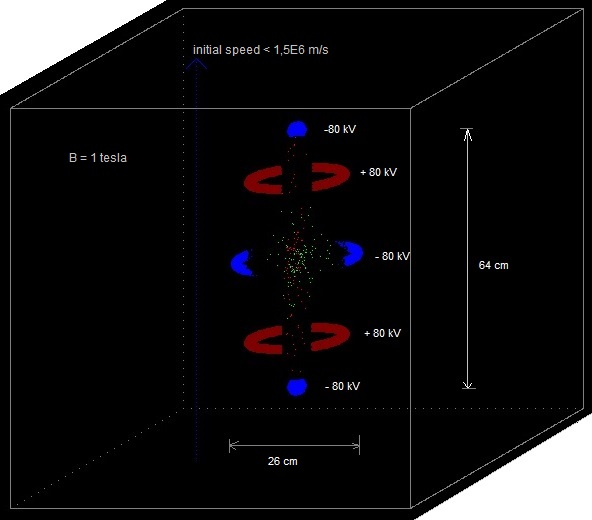Fig. 11. Reversed polarities and  less initial speed, allowing less voltages and smaller dimensions (exp. 11.20)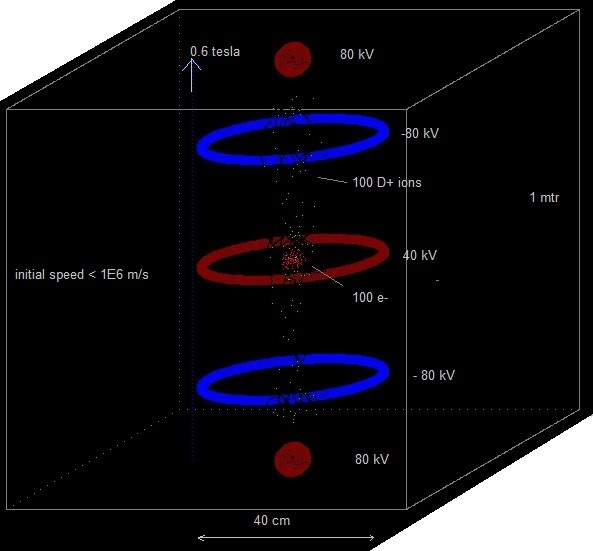Maybe we could use a small inner grid ( as in a Farnsworth fusor) to start the operation .

Fig. 12. SEM fusor with small inner starting grid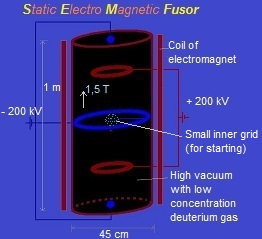What polarity is the best to be used we should investigate. (fig. 6 or fig. 7). We could construct an experimental SEM fusor with the possibility to reverse the polarities and try out.

Possible modus operandi:
Pump vacuum.
Let in a little bit deuterium gas. (Or inject deuterium ions? But then no inner starting grid will be necessary.)
Switch on the electromagnet.
Put on the voltages of the rings, small spheres and the inner grid.
The inner grid will burn, but an amount of D+ ions will have concentrated in the centre.
Inject electrons?  To form a virtual cathode? (for example as in fig. 9 or fig. 7)

Plenty of experimental work could be done to try, find out....

The big question: will fusion take place, as in the Farnsworth fusor?

Fig. 12. The electric field.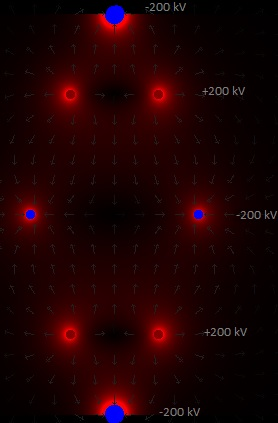The electric field can now be visualized,
see 11.5 Visualizing the electric field

---

The electrons represent the greatest loss and maximal heating component of the traditional fusor. They are accelerated just like the deuterium ions, but towards the shell wall. They slam into it producing x-rays and accounting for the bulk of the heating of the outer shell wall. See:
Fusor.net detailed theory of operation

In our SEM fusor the electrons stay also confined!

---

Is it possible to charge the rings and spheres with 200 kV?

Let's take a sphere with a radius of 1 cm:
Q = V.r/(9E9) = 200E3.0,01/(9E9) = 2.2E-7 C.
The electric field just near its surface:
E = 9E9.Q/r2 = 9E9.2,2E-7 /0,012 = 2E7 V/m = 200 kV/cm

In www.researchgate.net/  is mentioned "generally, for distances of about 1 cm and pressures of about 10^-7 torr the dielectric strength is ranging from 200 kV/cm to 400 kV/cm. Even larger values are possible for lower pressures".

"Field emission in pure metals occurs in high electric fields: the gradients are typically higher than 1 gigavolt per metre".

So it seems to be that we will not have problems with dielectric breakdown or field emission, if our fusor has a good vacuum.

---

Applying a mirror magnetic field to the SEM fusor and see if this will improve it.

Fig. 13. Applying a magnetic mirror field.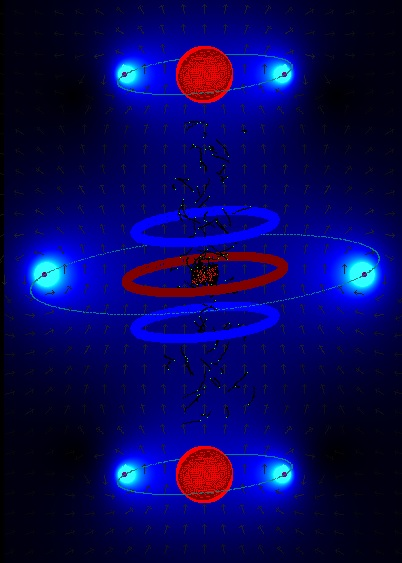By doing so we can decrease (a little bit) the voltages of the charged rings and spheres.

---

It is not possible to simulate more than a couple of hundred particles, because lack of computer power.
But let's see what happens if we both increase the mass and the charge of the ions and electrons.
See: Droom11.15.htm

This example demonstrates that we can not simply represent a group of particles by a few particles:

----

In alpha.web.cern.ch/penningtrap a device  a little bit simular to the SEM fusor is used, but not for fusion. Here the goal is to trap antiprotons and positrons in order to produce antihydrogen. According to them the electrons cool down due to cyclotron_radiation .  "Antiprotons, however, are far more massive. It would take antiprotons over 300 years of sitting in a 1 Tesla magnetic field to cool through cyclotron radiation alone!" That the electrons cool down in the SEM fusor is only favorable, I think. So they form even better a negative cloud: a virtual cathode (I suppose). The protons however should be hot, move with high speeds in order to be able to fuse.

--

"At the center of the Sun, fusion power is estimated by models to be about 276.5 watts/m3. Despite its intense temperature, the peak power generating density of the core overall is similar to an active compost heap, and is lower than the power density produced by the metabolism of an adult human."

This is not very promising...

---

Will fusion take place? (various calculations)

---

Fig. 14. Generating the D+ ions and the electrons all over the vacuum chamber,
but with zero speed.Some D+ ions obtain speeds till 2,3E6 m/s (more or less in the centre region).

---

An example that demonstrates that we cannot simply representate a group of particles by a few one:  Droom11.18.htm

---

Duinlab\Duin1.html ->  quite a different approach

---

If we inject and confine boron ions B+ , hydrogen ions H+ and electrons, perhaps we achieve the reaction:

115B + 11H  -> 3 42He  + 8,68 MeV

An advantage of this fusion reaction with boron is that it (almost) not produces neutrons, which are dangerous and noxious.

---

The simpler the design the better, I suppose..
Or maybe not better, but yes more beautiful.

The next simulation was with only two negatively charged rings:

Fig. 15.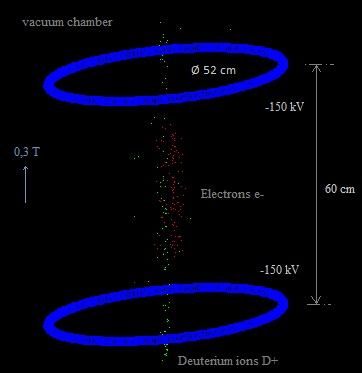Video

---

Accelerating D+ ions with a voltage difference of 900 V gives them a speed of 3. 105 m/s .

---

Latest experiments

---

An idea is perhaps a configuration with 6 rings:

Fig. 16.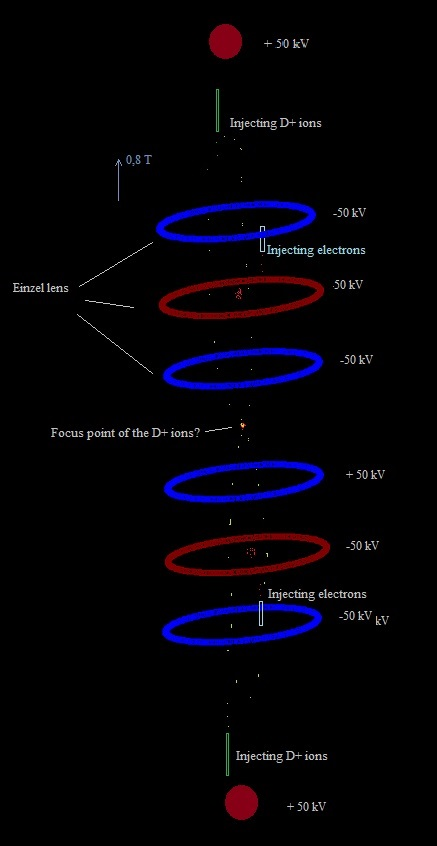Droom13.htm

---

An design that has perhaps some similarities with the SEM fusor:
Fenley patent.htm

---

A new idea (applying a kind of reversed magnetic bottle)

---

Will voltage breakdown occur in the Sem Fusor?

----

Injecting the electrons from the side, horizontally

The interaction of the magnetic and the electric field results in a bit strange movement of the electrons:

Fig. 17.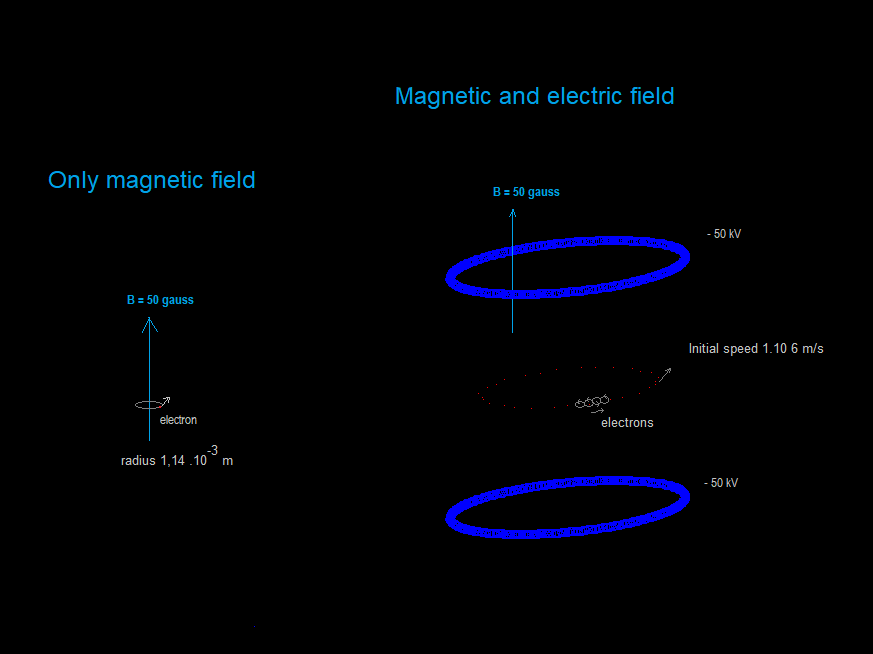With only a magnetic field they move in small circels around the vertical field lines. But if also an electric field is applied, produced by two negatively charged rings, they move also in a "big circle". And the radius of this big circle seems to be, more or less, independent of the strength of the magnetic field and the voltage of the rings. Quite curious..

Recording of various experiments in youtube

-----------------

Some more experiments with the configuration with only two negatively charged rings:

Fig. 18.When a kind of "Bremsstrahlung" is introduced in the program (reducing the speed of the electrons),
then the vertical component of the speed of the electrons decreases almost to zero and the electrons
concentrate in the centre, more or less in the horizontal plan. But only if there is a limit to the reducing.
If the simulation keeps reducing, then the electrons move slowly to the sides in the horizontal centre plane,
and eventually escape. In reality the electrons will never stop moving, I suppose. They will always receive
some energy from their environment (heat radiation, colisions with other particles). So hopefully, in a real experiment,
the electrons will concentrate in the centre, and form a kind of virtual negative electrode which will atract the D+ ions
more to the centre and possible cause them to fuse, as in a Farnsworth-Hirsch fusor.

----------------------

A first experiment to do could be:
A vacuumchamber with a little D2 gas.
Two rings, to be charged with about -100 or - 150 kV.
An electron gun to inject electrons just above the lower ring.
An magnetic field of 0,4 T (with a permanent magnet of an used rmi scanner?).
Then see if a elecron cloud is formed in the centre, and if D+ ions are atracted to this electron cloud.
If we measure that neutrons are produced, then this would be a sign that fusion takes place.
But probably many D+ ions will collide with the negative charged rings.

Better would be to inject also the D+ ions into the vacuum chamber. See also Droom15.htm#Conclusion, so far:

Or perhaps we inject a neutral beam from the top and from the bottom which collides in the centre, where D+ and electrons are formed:

Droom16.htm (simulation generating the D+ and electrons in the centre)

-----------------------------------------

Exp. 11.9.7. Two negatively charged rings, D+ and e- injected from below to up.
Magnetic bottle field generated by three current carying loops.
Speed electrons decreased simulating Bremsstrahlung.
The D+ ions stay confined, and a little squezed in the centre region.

-----------------------<
Forums:
Discussion in Fusor.net

Scienceforms.net (silence..)
Some discussion in Reddit.com
------

Conclusion so far:

The Sem Fusor design will not be so easy to make, with its high voltages, magnetic field of 0,5 till 1,5 telsa, vacuumchamber, injecting the deuterium ions and electrons or a neutral beam... But it should be possible, with sufficient funds.

Doubts: How many electrons will be confined in the centre? Will they form a virtual negative electrode, which could atract the positive deuterium ions? Will they neutralize with D+ ions? Will the deuterium ions sufficiently be confined by the magnetic field, the charged rings and the electron cloud in the centre?  And will some of them obtain such high speeds so that fusion can take place? A bit similar as in a Farnsworth Fusor ? And if fusion occurs, will there be a net energy gain?

The simulation program only demonstrates that we can confine a couple of hundred of deuterium ions and electrons in a static electric and magnetic field. But it would be very interesting to perform real systematic experiments.

With about 2 millions (dollar or euro) we could buy a building for a lab, magnet, instruments... and do real experiments.

The fusion experiment ITER in the south of France costs about 20000 millions... so 2 millions isn´t so much J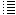20 February 2014-..     by  Rinze Joustra        www.valgetal.com